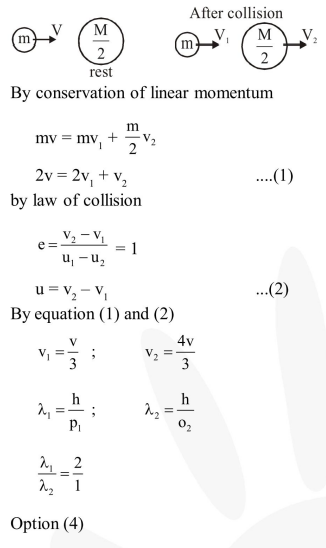Deepak Scored 45->99%ile with Bounce Back Crack Course. You can do it too!

# A particle A of mass m and initial velocity v collides with a particle

Question:

A particle A of mass $m$ and initial velocity $v$ collides with a particle $B$ of mass $\frac{m}{2}$ which is at rest. The collision is head on, and elastic. The ratio of the de-Broglie wavelengths $\lambda_{\mathrm{A}}$ to $\lambda_{\mathrm{B}}$ after the collision is :

1. $\frac{\lambda_{\mathrm{A}}}{\lambda_{\mathrm{B}}}=\frac{2}{3}$

2. $\frac{\lambda_{\mathrm{A}}}{\lambda_{\mathrm{B}}}=\frac{1}{2}$

3. $\frac{\lambda_{\mathrm{A}}}{\lambda_{\mathrm{B}}}=\frac{1}{3}$

4. $\frac{\lambda_{\mathrm{A}}}{\lambda_{\mathrm{B}}}=2$

Correct Option: , 4

Solution: